A Gaussian Quadrature-like formula for numerical estimation of integrals. It requirespoints and fits all Polynomials to degree, so it effectively fits exactly all Polynomials of degree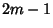. It uses a Weighting Functionin which the endpointin the interval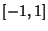is included in a total ofAbscissas, givingfree abscissas. The general formula is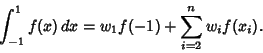(1)

The free abscissasfor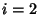, ...,are the roots of the Polynomial(2)

whereis a Legendre Polynomial. The weights of the free abscissas are(3)

and of the endpoint(4)

The error term is given by(5)

for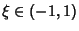.20.5 0.333333 1.5 30.2222221.02497 0.689898 0.752806 40.1250.657689 0.181066 0.776387 0.822824 0.440924 50.080.4462080.623653 0.446314 0.562712 0.885792 0.287427

The Abscissas and weights can be computed analytically for small.2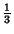3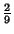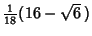References

Abramowitz, M. and Stegun, C. A. (Eds.). Handbook of Mathematical Functions with Formulas, Graphs, and Mathematical Tables, 9th printing. New York: Dover, p. 888, 1972.

Chandrasekhar, S. Radiative Transfer. New York: Dover, p. 61, 1960.

Hildebrand, F. B. Introduction to Numerical Analysis. New York: McGraw-Hill, pp. 338-343, 1956.